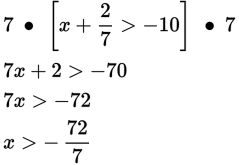# SAT Math Multiple Choice Question 325: Answer and Explanation

### Test Information

Question: 325

10. Which of the following represents the solution set for the inequality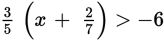?

• A.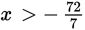• B.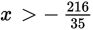• C.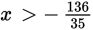• D.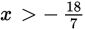Explanation:

A

Difficulty: Medium

Category: Heart of Algebra / Inequalities

Strategic Advice: When an equation or an inequality involves fractions, there are a number of ways to approach it. You could distribute the fractions or you could clear the fractions by multiplying both sides by the lowest common denominator. In this question, clearing one fraction at a time will prevent having to work with messy fractions and large numbers.

Getting to the Answer: Solve the inequality one step at a time. First, multiply everything by 5, and then divide by 3-this will clear the first fraction: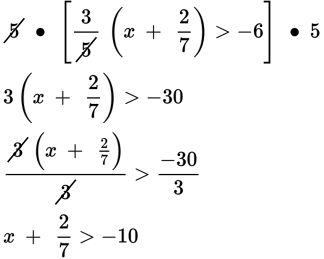Now, multiply everything by 7 and go from there: